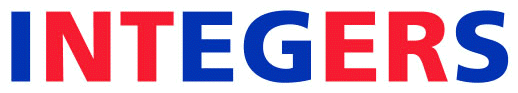Formatting your paper for INTEGERS: LaTeX

Important:  Please include any and all line skips
that are given below.



\documentclass[10pt]{article}
\textwidth= 5.00in
\textheight= 7.4in
\topmargin = 30pt
\evensidemargin=0pt
\oddsidemargin=55pt
\parskip=.5pt
\parindent=12pt
\font\smallit=cmti10
\font\smalltt=cmtt10
\font\smallrm=cmr9

\usepackage{amssymb,latexsym,amsmath,epsfig,amsthm} %% Add other packages as necessary

\makeatletter

\renewcommand\section{\@startsection {section}{1}{\z@}
{-30pt \@plus -1ex \@minus -.2ex}
{2.3ex \@plus.2ex}
{\normalfont\normalsize\bfseries\boldmath}}

\renewcommand\subsection{\@startsection{subsection}{2}{\z@}
{-3.25ex\@plus -1ex \@minus -.2ex}
{1.5ex \@plus .2ex}
{\normalfont\normalsize\bfseries\boldmath}}

\renewcommand{\@seccntformat}{\csname the#1\endcsname. }

\newcommand\blfootnote{
\begingroup
\renewcommand\thefootnote{}\footnote{#1}
\endgroup
}

\makeatother

\newtheorem{theorem}{Theorem}
\newtheorem{lemma}{Lemma}
\newtheorem{proposition}{Proposition}
\newtheorem{corollary}{Corollary}

\theoremstyle{definition}
\newtheorem{definition}{Definition}
\newtheorem{conjecture}{Conjecture}
\newtheorem{remark}{Remark}
\newtheorem{example}{Example}

%% add any other theorem environments you will used

\begin{document}

Next comes your title and author list information in the
following construct:



\begin{center}
\vskip 20pt
{\bf Author One\footnote{any footnote here}}\\
{\smallit Department of Mathematics, One University, Somewhere, State, Country}\\
{\tt me@math.one.edu}\\ (optional)
\vskip 10pt
{\bf Author Two\footnote{any footnote here}}\\
{\smallit Department of Mathematics, University of Two, Anywhere, Country}\\
{\tt you@math.two.edu}\\ (optional)
\end{center}
\vskip 20pt

\centerline{\smallit Received: , Revised: , Accepted: , Published: } % We will fill in the dates
\vskip 30pt



Followed by your abstract in the following construct:


\centerline{\bf Abstract}

\noindent
Put your abstract here. Please limit it to half of a page of text.

\markright{\smalltt INTEGERS: 23 (2023)\hfill}
\thispagestyle{empty}
\baselineskip=12.875pt
\vskip 30pt





SOME IMPORTANT GUIDELINES TO FOLLOW

• Do not include zip codes or postal numbers in authors' affiliations.

• All sentences should begin with an English word. Do not begin a sentence with mathematical notation

• All statements, including those used to introduce a Theorem, should be complete sentences

• Do not use contractions (e.g., that's, we'd, don't).

• When giving definitions, use italics (but not boldface) for the words being defined.

• If you state a numbered theorem or other result that is cited from a previous publication, please format the first instance as in the following example:

\noindent{\bf Theorem 1} (). {\em If $x > 1$, then $x+1 > 2$.}
or
\begin{theorem}[\cite{XYZ}] If $x > 1$, then $x+1 > 2$.
\end{theorem}

• Table labels should appear just beneath the table (not above).

• When referring to a numbered theorem or other result, capitalize the word "Theorem" (or Lemma, Corollary, etc.), and do not abbreviate the word.

• When referring to a numbered equation in the text, write it in the form "Equation (n)" where n is the number of the equation

• Do not use the symbols "&", "\exists" or "\forall," but instead write the words, i.e., "and", "there exists," and "for all."

• Whenever practical, when using logical connectives, such as "if and only if" and "implies," use words rather than arrows or double arrows.

• Please denote the start of your proofs by \begin{proof} and end them with \end{proof}

• Acknowledgement Section: If used, it should be at the end of the paper, before the bibliography. It should be formatted in regular font, and should begin with \noindent {\bf Acknowledgement.} or \noindent {\bf Acknowledgements.}

• REFERENCES: For references, please list them alphabetically by first author's last name and have the labels be numeric.

For article titles, only the first word and proper nouns should be capitalized. For book titles, all words, except articles, prepositions, and conjunctions, should be capitalized.

It is important that your references be part of your paper and not in a separate file. The Reference section should be the last section, unless there are appendices (which come after the reference section).

J. Deer and K. Doe, On the history of mathematics, {\it J. of the World} {\bf 52} (1999), 123-135.

A. Jones, L. Smith, and C. Vector, {\it The Theory of Everything}, Publishing Company, New York, 1987.

• For papers appearing within larger works (e.g., a paper in a conference proceedings, a book chapter, etc.), please follow the following format:

A. Reid and B. Wright, Important number theory result, in {\it The Big Math Book}, Publishing Company, Boston, MA, 1975.

R. Evans and D. Zager, Math in the future, preprint, {\tt arXiv: 2525.31415}.

• Your reference section should be of the following form:

\begin{thebibliography}{1}\footnotesize

\bibitem{A} J. Author, My first math paper, {\it Integers} {\bf 25} (2020), \#A32.

\bibitem{CD} I. Can and N. Do, Proof of existence, {\it J. Math. Stuff} {\bf 17}(2000), 19-23.

\end{thebibliography}

• If you use BibTeX, Peter Selinger has provided a BibTex style file, which formats according to our journal standards. The only item to address with its usage is to use the abbreviated names of journals. The editors at Integers do not make any warranty on this and cannot provide any troubleshooting on its usage.